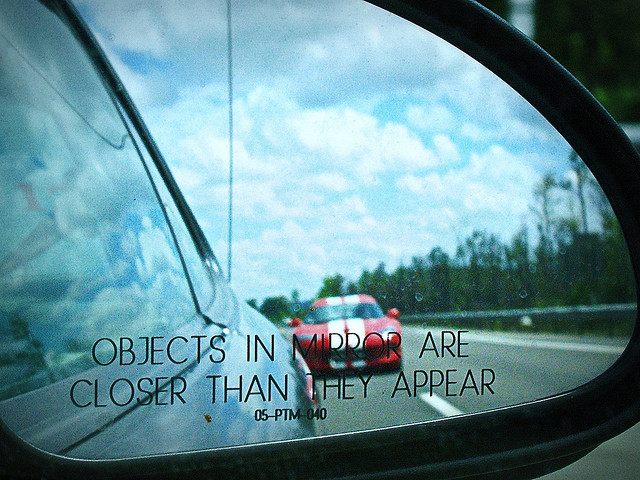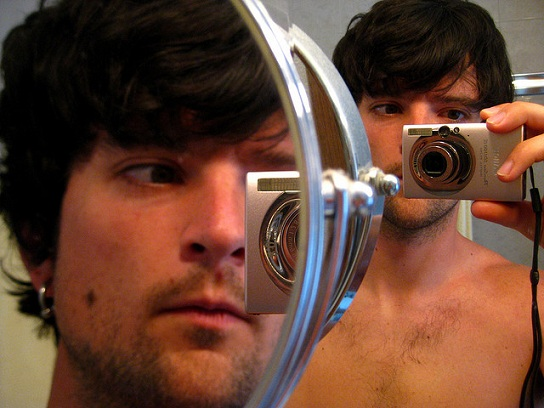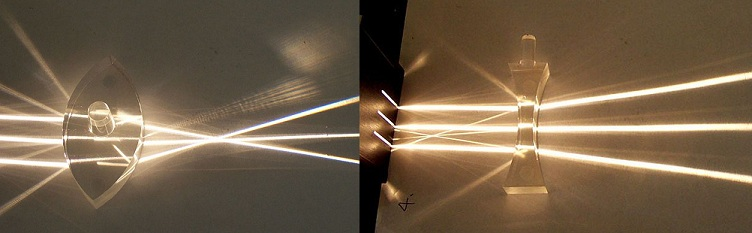# Difference Between Mirror and Lens

## Main Difference – Mirror vs. Lens

Mirrors and lenses are used ubiquitously to manipulate light in order to produce images of objects according to our needs. The main difference between mirror and lens is that a mirror works by reflecting light, whereas a lens works by refracting light.

## What is a Mirror

A mirror is most often made with a highly polished surface of a metal, placed behind a glass. A mirror works by reflecting light that falls on it according to the law of reflection. This law states that when a ray of light is incident on a surface:

1. The angle of incidence is equal to the angle of reflection.
2. The incident ray, the normal and the reflected ray all lie in the same plane.

The diagram below illustrates this law for a plane mirror. Here, PO is the incident ray and OQ is the reflected ray.Law of Reflection: The angle of incidence is equal to the angle of reflection

When the surface of the mirror is curved, things become more interesting. A convex mirror is one that curves outwards. It produces diminished, upright, virtual images of objects placed in front of it. These types of mirrors are used in vehicles to give the driver a larger field of view.Cars use convex mirrors. Hence, “objects in mirror are closer than they appear”.

Concave mirrors curve inward. The nature of the image formed by a concave mirror depends on where the object is positioned in front of the mirror. Virtual, upright, enlarged images can be observed if the object is placed between the mirror and its principle of focus. Mouth mirrors used by dentists are concave, used to produce a magnified image of patient’s teeth. Often, concave mirrors are also used to magnify a person’s face while shaving.A concave mirror can produce a magnified image. Compare with the unmagnified image produced by the plane mirror (in the background)

The nature of images produced by mirrors can be computed using the mirror equation.

## What is a Lens

A lens is a transparent object that transmits light across it, and its working principle depends on the law of refraction (Snell’s law). This law describes, by how much a ray of light bends when it passes from one medium to another, depending on the refractive indexes of the two media. Different types of lenses can be formed by combining flat, convex (curving outward) and concave (curving inward) surfaces. Convex surfaces converge rays of light passing through it, whereas concave surfaces diverge light rays passing through it. The image below shows how rays of light are refracted as they pass through a biconvex (left) and a biconcave lens (right).Different types of lenses: the biconvex lens on the left converges rays of light passing through it while the biconcave lens on the right diverges rays of light passing through it.

The precise nature of the image formed by a lens depends not only on the nature of the lens but also on where the object is placed. For instance, a magnifying glass (which is a biconvex lens) can be held close to an object to view a virtual, enlarged and upright image of the object, while the same lens can be used to project a real, inverted and diminished image of an object placed far away. The nature of the image produced by mirrors can be calculated using the lens equation.

Lenses are commonly used to correct defects in vision. For instance, concave lenses can be used to correct short-sight while convex lenses can be used to correct long-sight.

## Difference Between Mirror and Lens

### Working Principle

Mirrors work by reflecting light incident on them.

Lenses work by refracting light passing through them.

### Composition

mirror typically consists of a highly polished surface of a metal placed behind a glass.

lens is typically a bent piece of glass or plastic.

### Calculations

Calculations involving the nature of images formed by mirrors is done using the mirror equation.

Calculations involving the nature of images formed by lenses is done using the lens equation.

Image courtesy:

“Angle of incidence equals the angle of reflection on a mirror” by Johan Arvelius (Own work) [CC BY-SA 3.0], via Wikimedia Commons

“Objects in Mirror” by Shawn Carpenter (Own work) [CC BY-SA 2.0], via flickr

“Denver: Magnifying Mirror” by Eli Duke (Own work) [CC BY-SA 2.0], via flickr

“Convex lens” by User Fir0002 on en.wikipedia (http://en.wikipedia.org/wiki/Image:Large_convex_lens.jpg) [CC BY-SA 3.0], via Wikimedia Commons (modified)

“Concave lens” by User Fir0002 on en.wikipedia (Originally from en.wikipedia; description page is (was) here) [CC BY-SA 3.0], via Wikimedia Commons (modified)